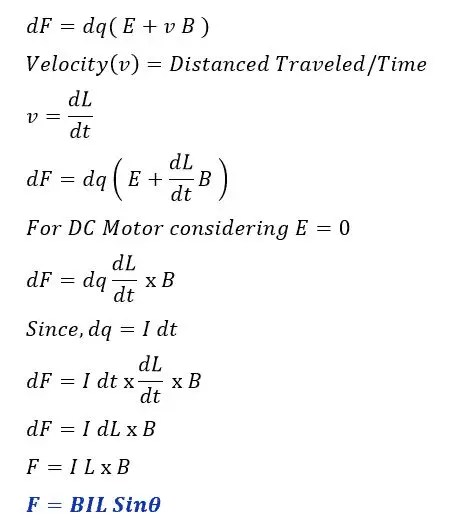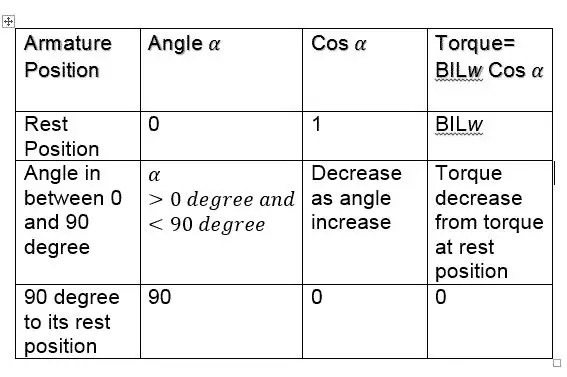# Working or Operating Principle of DC Motor

The DC motor converts electrical energy into mechanical energy. The input power to DC motor is DC power. The DC motor works on the principle of Lorenz law which states that when the current carrying conductor is placed in a magnetic field, it experiences a force. The force is called the Lorenz force and its direction can be found by Fleming’s left hand rule.

## Working Principle of DC Motor

The DC motor has armature and field winding. The armature is connected to the DC supply source through commutator segment and carbon brushes. The armature is placed under the magnetic field. The magnetic field is produced in the DC motor when its field winding is energized with DC supply.

The single loop construction of DC motor is as given below.The DC motor has armature and field winding. The armature is connected to the DC supply source through commutator segment and carbon brushes. The armature is placed under the magnetic field. The magnetic field is produced in the DC motor when its field winding is energized with DC supply.

When the current carrying armature conductor is placed under the magnetic field the conductor experience a force. The direction of the force experienced by conductor can be determined by Fleming’s left hand rule.

When the current carrying armature conductor is placed under the magnetic field the conductor experience a force. The direction of the force experienced by conductor can be determined by Fleming’s left hand rule.According to the Fleming’s left hand rule, if we extend the index finger, middle finger and thumb of our left hand in such a way that middle finger is in the direction of current in the conductor, and index finger is along the direction of magnetic field, i.e., north to south pole,then thumb indicates the direction of the created mechanical force.

Magnitude of Force

When infinitely small charge dq is made to flow at velocity of v under the electric field E and magnetic field B, then the Lorenz force experienced by charge dq is given by;In DC motor, the direction of  current through the armature conductor  at all instant is  perpendicular to the field. The current in the left hand side of the coil is I and current in right side coil is -I.The force acting on the left hand side coil is;
F = BIL Sinθ
θ = 90

F = BIL Sin90  [ Sin 90 = 1 ]
F= BIL
The force acting on the right hand side coil is;
Fr = BIL Sinθ
θ = 90

Fr = B(-I )L Sin90  [ Sin 90 = 1 ]
Fr = – BIL

In DC motor, the direction of  current through the armature conductor  at all instant is  perpendicular to the field. The current in the left hand side of the coil is I and current in right side coil is -I.

Thus the equal magnitude of the force is developed at the two sides of the coil.However, the direction of both the forces is opposite to each other. The opposite force acting on the two sides of a coil which are  distance apart by ‘w’ cause rotation of the coil and the coil starts rotating.In this way, rotational torque is produced in DC motor.

## Magnitude of Torque

The torque of DC motorThe torque produced by DC motor depends  on the angle between the plane of the armature turn and plane of reference. When armature is at rest position, it produce maximum torque and the torque reduces with increase of angle.Related Posts

1. Types of Braking in a DC Motor
2. What is Plugging or Reverse Current Braking of DC Motor?
3. Speed Regulation of DC Motor | Definition and Formula